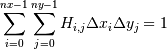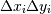numpy.histogram2d¶

numpy.histogram2d(x, y, bins=10, range=None, normed=False, weights=None)[source]

Compute the bi-dimensional histogram of two data samples.

Parameters : x : array_like, shape (N,) An array containing the x coordinates of the points to be histogrammed. y : array_like, shape (N,) An array containing the y coordinates of the points to be histogrammed. bins : int or [int, int] or array_like or [array, array], optional The bin specification: If int, the number of bins for the two dimensions (nx=ny=bins). If [int, int], the number of bins in each dimension (nx, ny = bins). If array_like, the bin edges for the two dimensions (x_edges=y_edges=bins). If [array, array], the bin edges in each dimension (x_edges, y_edges = bins). range : array_like, shape(2,2), optional The leftmost and rightmost edges of the bins along each dimension (if not specified explicitly in the bins parameters): [[xmin, xmax], [ymin, ymax]]. All values outside of this range will be considered outliers and not tallied in the histogram. normed : bool, optional If False, returns the number of samples in each bin. If True, returns the bin density, i.e. the bin count divided by the bin area. weights : array_like, shape(N,), optional An array of values w_i weighing each sample (x_i, y_i). Weights are normalized to 1 if normed is True. If normed is False, the values of the returned histogram are equal to the sum of the weights belonging to the samples falling into each bin. H : ndarray, shape(nx, ny) The bi-dimensional histogram of samples x and y. Values in x are histogrammed along the first dimension and values in y are histogrammed along the second dimension. xedges : ndarray, shape(nx,) The bin edges along the first dimension. yedges : ndarray, shape(ny,) The bin edges along the second dimension.

histogram
1D histogram
histogramdd
Multidimensional histogram

Notes

When normed is True, then the returned histogram is the sample density, defined such that:where H is the histogram array andthe area of bin {i,j}.

Please note that the histogram does not follow the Cartesian convention where x values are on the abcissa and y values on the ordinate axis. Rather, x is histogrammed along the first dimension of the array (vertical), and y along the second dimension of the array (horizontal). This ensures compatibility with histogramdd.

Examples

>>> import matplotlib as mpl
>>> import matplotlib.pyplot as plt

Construct a 2D-histogram with variable bin width. First define the bin edges:

>>> xedges = [0, 1, 1.5, 3, 5]
>>> yedges = [0, 2, 3, 4, 6]

Next we create a histogram H with random bin content:

>>> x = np.random.normal(3, 1, 100)
>>> y = np.random.normal(1, 1, 100)
>>> H, xedges, yedges = np.histogram2d(y, x, bins=(xedges, yedges))

Or we fill the histogram H with a determined bin content:

>>> H = np.ones((4, 4)).cumsum().reshape(4, 4)
>>> print H[::-1]  # This shows the bin content in the order as plotted
[[ 13.  14.  15.  16.]
[  9.  10.  11.  12.]
[  5.   6.   7.   8.]
[  1.   2.   3.   4.]]

Imshow can only do an equidistant representation of bins:

>>> fig = plt.figure(figsize=(7, 3))
>>> ax.set_title('imshow:
equidistant’)
>>> im = plt.imshow(H, interpolation='nearest', origin='low',
extent=[xedges, xedges[-1], yedges, yedges[-1]])

pcolormesh can displaying exact bin edges:

>>> ax.set_title('pcolormesh:
exact bin edges’)
>>> X, Y = np.meshgrid(xedges, yedges)
>>> ax.pcolormesh(X, Y, H)
>>> ax.set_aspect('equal')

NonUniformImage displays exact bin edges with interpolation:

>>> ax.set_title('NonUniformImage:
interpolated’)
>>> im = mpl.image.NonUniformImage(ax, interpolation='bilinear')
>>> xcenters = xedges[:-1] + 0.5 * (xedges[1:] - xedges[:-1])
>>> ycenters = yedges[:-1] + 0.5 * (yedges[1:] - yedges[:-1])
>>> im.set_data(xcenters, ycenters, H)
>>> ax.images.append(im)
>>> ax.set_xlim(xedges, xedges[-1])
>>> ax.set_ylim(yedges, yedges[-1])
>>> ax.set_aspect('equal')
>>> plt.show()

numpy.histogram

Next topic

numpy.histogramdd